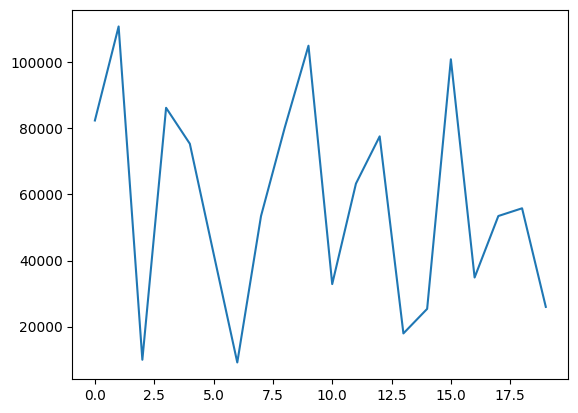# 2.7 Data analysis with classes#

Estimated time to complete this notebook: 10 minutes

Earlier, we wrote some code to measure the amount of green content on satellite images. Now, we’re going to convert this into a “Greengraph” class, and save it as a module.

⚠️ It is generally a better idea to create files in an editor or integrated development environment (IDE) rather than through the notebook! ⚠️

## 2.7.1 Classes for Greengraph#

%%bash
mkdir -p greengraph  # Create the folder for the module (on mac or linux)

%%writefile greengraph/graph.py
import numpy as np
import geopy
from .map import Map

class Greengraph:
def __init__(self, start, end):
self.start = start
self.end = end
self.geocoder = geopy.geocoders.Nominatim(user_agent="rsd-course")

def geolocate(self, place):
return self.geocoder.geocode(place, exactly_one=False)

def location_sequence(self, start, end, steps):
lats = np.linspace(start, end, steps)
longs = np.linspace(start, end, steps)
return np.vstack([lats, longs]).transpose()

def green_between(self, steps):
return [
Map(*location).count_green()
for location in self.location_sequence(
self.geolocate(self.start), self.geolocate(self.end), steps
)
]

Overwriting greengraph/graph.py


Note that a line like from .map import Map will import the definition of Map from the file map.py in the current directory.

%%writefile greengraph/map.py

import numpy as np
from io import BytesIO
import imageio as img
import requests

class Map:
def __init__(
self, lat, long, satellite=True, zoom=10, size=(400, 400), sensor=False
):
base = "https://static-maps.yandex.ru/1.x/?"

params = dict(
z=zoom,
size=str(size) + "," + str(size),
ll=str(long) + "," + str(lat),
l="sat" if satellite else "map",
lang="en_US",
)

self.image = requests.get(
base, params=params
).content  # Fetch our PNG image data
content = BytesIO(self.image)
self.pixels = img.imread(content)  # Parse our PNG image as a numpy array

def green(self, threshold):
# Use NumPy to build an element-by-element logical array
greener_than_red = self.pixels[:, :, 1] > threshold * self.pixels[:, :, 0]
greener_than_blue = self.pixels[:, :, 1] > threshold * self.pixels[:, :, 2]
green = np.logical_and(greener_than_red, greener_than_blue)
return green

def count_green(self, threshold=1.1):
return np.sum(self.green(threshold))

def show_green(data, threshold=1.1):
green = self.green(threshold)
out = green[:, :, np.newaxis] * array([0, 1, 0])[np.newaxis, np.newaxis, :]
buffer = BytesIO()
result = img.imwrite(buffer, out, format="png")
return buffer.getvalue()

Overwriting greengraph/map.py

%%writefile greengraph/__init__.py
from .graph import Greengraph

Overwriting greengraph/__init__.py


## 2.7.2 Invoking our code and making a plot#

%matplotlib inline
from greengraph import Greengraph
from matplotlib import pyplot as plt

mygraph = Greengraph("New York", "Chicago")
data = mygraph.green_between(20)

plt.plot(data)

[<matplotlib.lines.Line2D at 0x7fce7878a4f0>]